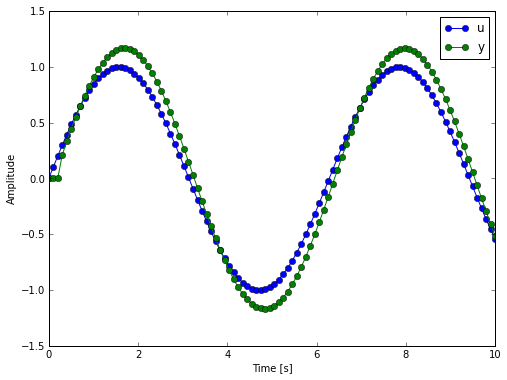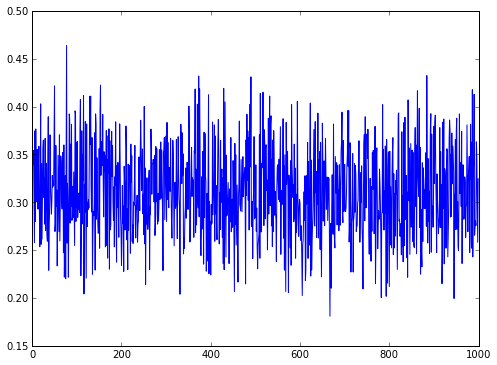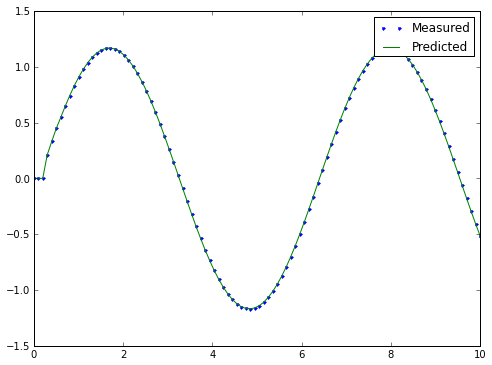In :
%pylab inline
rcParams['figure.figsize'] = (8, 6)

Populating the interactive namespace from numpy and matplotlib


# Section 1.1¶

In :
import numpy as np
from numpy.testing import assert_allclose


$n$ is the number of past outputs and $m$ is the number of past inputs to use when predicting $y(t)$. Then I form the some random arrays to use as example coefficients, I will identify them later. $\theta$ are the parameters I wish to identify.

In :
n = 3
m = 2

a = 0.1 * np.random.random(n)
b = np.random.random(m)

theta = np.hstack((a, b))
theta

Out:
array([ 0.0788782 ,  0.04282267,  0.0912454 ,  0.72957521,  0.68514357])

Define $u(t)$ as the known input and $y(t)$ as the output which is a function of $\theta$. I choose $u(t)$ but compute $y(t)$. Notice that we can't compute $y(t)$ for at least the first $n$, because there is not enough past information. $N$ is the number of time steps. It is typical to set values outside the measured range to zero.

In :
N = 100
time = np.linspace(0.0, 10.0, num=N)
u = np.sin(time)
y = np.hstack((np.zeros(n), np.array((N - n) * [np.nan], dtype=float)))

for i in range(len(u)):
if np.isnan(y[i]):
previous_outputs = -a * y[i - n:i]
previous_inputs = b * u[i - m:i]
y[i] = previous_outputs.sum() + previous_inputs.sum()

In :
plot(time, u, '-o', time, y, '-o')
xlabel("Time [s]")
ylabel('Amplitude')
legend(['u', 'y'])

Out:
<matplotlib.legend.Legend at 0x3b9d750>Now construct $\varphi(t)$, the regressor. $\varphi(t)$ is a vector, but we can store is in a 2D array with the rows indexing time and the columns indexing past values.

In :
phi = np.zeros((len(u), len(a) + len(b)))
for i, phi_of_t in enumerate(phi):
if i >= n:
phi[i] = np.hstack((-y[i - n:i], u[i - m:i]))
assert_allclose(np.sum(phi[i] * theta), y[i])
phi[:10]

Out:
array([[ 0.        ,  0.        ,  0.        ,  0.        ,  0.        ],
[ 0.        ,  0.        ,  0.        ,  0.        ,  0.        ],
[ 0.        ,  0.        ,  0.        ,  0.        ,  0.        ],
[-0.        , -0.        , -0.        ,  0.10083842,  0.20064886],
[-0.        , -0.        , -0.21104249,  0.20064886,  0.2984138 ],
[-0.        , -0.21104249, -0.33158808,  0.2984138 ,  0.39313661],
[-0.21104249, -0.33158808, -0.44777705,  0.39313661,  0.48385164],
[-0.33158808, -0.44777705, -0.54662684,  0.48385164,  0.56963411],
[-0.44777705, -0.54662684, -0.64808005,  0.56963411,  0.64960951],
[-0.54662684, -0.64808005, -0.74280452,  0.64960951,  0.72296256]])

The cost function that needs to be minimized with respect to $\theta$ looks like this:

In :
def VN(theta, y, phi):
square_of_residuals = np.zeros(len(y))
for i, y in enumerate(y):
square_of_residuals = (y - phi[i] * theta) ** 2
return mean(square_of_residuals)


If we gave if enough random values of theta, we should hit close to the solution, but the number of guesses should be really huge.

In :
num_of = 1000
random_thetas = np.random.random((num_of, len(theta)))

In :
cost = np.zeros(num_of)
for i, rand_theta in enumerate(random_thetas):
cost[i] = VN(rand_theta, y, phi)

In :
plot(cost)

Out:
[<matplotlib.lines.Line2D at 0x3cdbe50>]In :
random_thetas[np.argmin(cost)]

Out:
array([  1.99968616e-01,   6.79448703e-02,   8.47582235e-04,
8.84261046e-01,   8.85445505e-01])
$$A = \sum^N_{t=1}\varphi(t)^T \varphi(t)$$$$b = \sum^N_{t=1}\varphi(t)y(t)$$

$A$ is $d \times d$ where $d$ is len(theta).

In :
A = 0.0
b = 0.0
for i in range(N):
A += np.outer(phi[i], phi[i])
b += phi[i] * y[i]

In :
print("A is: \n{}".format(A))
print("b is: \n{}".format(b))

A is:
[[ 63.77829377  63.46928621  62.51237159 -53.27531814 -51.81740578]
[ 63.46928621  63.86476889  63.5888684  -54.29733856 -53.40959994]
[ 62.51237159  63.5888684   64.03013321 -54.77981424 -54.48303025]
[-53.27531814 -54.29733856 -54.77981424  46.89165318  46.73588252]
[-51.81740578 -53.40959994 -54.48303025  46.73588252  47.09000259]]
b is:
[-60.91810647 -62.66384178 -63.79832907  54.70595313  55.01502282]


This is a simple linear system with an exact non-trivial solution:

In :
theta_hat = np.dot(np.linalg.inv(A), b)
theta_hat

Out:
array([ 0.0788782 ,  0.04282267,  0.0912454 ,  0.72957521,  0.68514357])
In :
np.linalg.solve(A, b)

Out:
array([ 0.0788782 ,  0.04282267,  0.0912454 ,  0.72957521,  0.68514357])
In :
np.testing.assert_allclose(theta, theta_hat)

In :
plot(time, y, '.', time, np.sum(phi * theta_hat, axis=1))
legend(['Measured', 'Predicted'])

Out:
<matplotlib.legend.Legend at 0x3cc8a50>